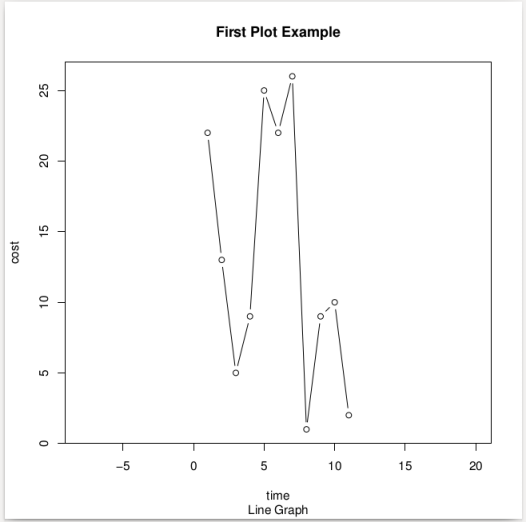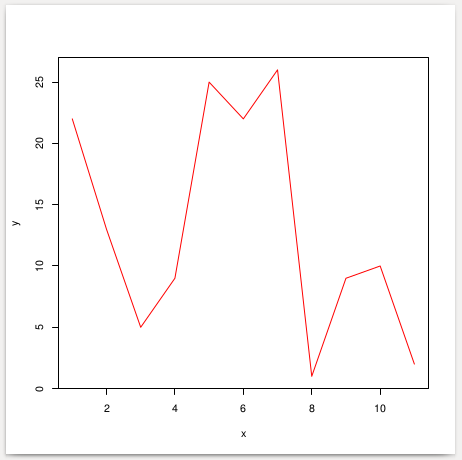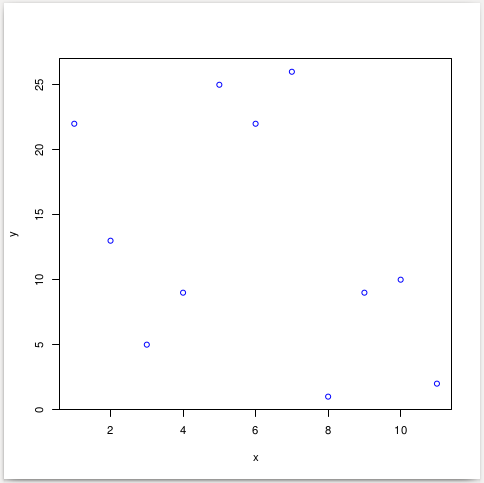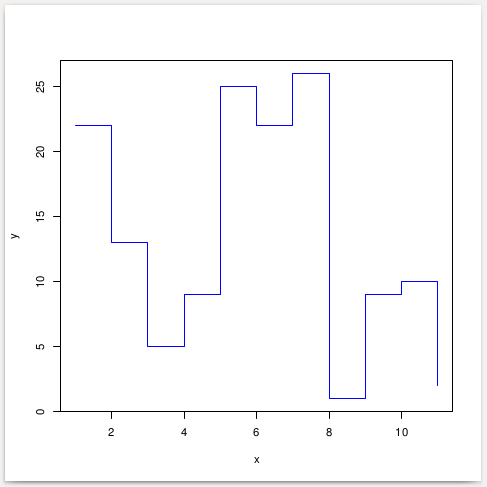Line Graph represents relation between two variables.

## Plot a line graph in R

We shall learn to plot a line graph in R programming language with the help of plot() function.

### Syntax of plot() function

where

• x is any R object with a plot method. Ex : numeric vector
• y is any R object with a plot method. Ex : numeric vector
• … is the extra arguments that could be provided, which may contain any of the following
• type  – type could be any of the below values
• ‘p’ – points
• ‘l’ – lines
• ‘b’ – both points and lines
• ‘c’ – for the lines part alone of both points and lines
• ‘o’ – for both points and lines overplotted
• ‘h’ – generates kind of histogram view
• ‘s’ – for stair step look
• ‘S’ – other steps
• ‘n’ – no plotting
• main is the main title for the plot
• sub is the sub title for the plot
• xlab is the x label i.e., title for x-axis
• ylab is the y label i.e., title for y-axis
• asp is the aspect ratio whose value should be given by y/x
• lwd is the line width
• pch is the point character. There are 25 symbols to choose from including alphabetic characters
• col gives the color to points and line

### Example 1 : Line Graph

When the above program is run, plot would be generated as a pdf file, by default.### Example 2 : Line, No points, Colored line

Output  Line Graph Plot### Example 3 : Points, No Line, Colored points

Output  Point Graph Plot### Example 4 : Stair Step Graph

Output  Stair Graph Plot## Conclusion

In this R Tutorial, we have leaned R plot function and some of the examples like plotting with both line and points, coloring the graph, drawing only points or lines on to the graph, etc.